American Journal of Theoretical and Applied Statistics
Volume 4, Issue 3, May 2015, Pages: 150-155

An Alternative Method of Estimation of SUR Model

Shohel Rana*, Mohammad Mastak Al Amin

Department of Mathematics and Natural Sciences, BRAC University, Dhaka, Bangladesh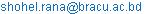(S. Rana)

Shohel Rana, Mohammad Mastak Al Amin. An Alternative Method of Estimation of SUR Model. American Journal of Theoretical and Applied Statistics. Vol. 4, No. 3, 2015, pp. 150-155. doi: 10.11648/j.ajtas.20150403.20

Abstract: This paper proposed a transformed method of SUR model which provided unbiased estimation in case of two and three equations of high and low co-linearity for both small and large datasets. The generalized least squares (GLS) method for estimation of seemingly unrelated regression (SUR) model proposed by Zellner (1962), Srivastava and Giles (1987),provided higher MSE. Although the Ridge estimators proposed by Alkhamisi and Shukur (2008) provided smaller MSE in comparison with others, it was not unbiased in case of severe multicollinearity.This study showed that our proposed method typically provided unbiasedestimator with lower MSE and TMSE than traditional methods.

Keywords: SUR Model, GLS, MSE, TMSE

Contents

1. Introduction

A set of equations that might be related not because of they interact, but also their error terms were related.A seemingly unrelated regression (SUR) system comprises several individual relationships that were linked by the fact that their disturbances were correlated. There were two main motivations for use of SUR. The first one was to gain efficiency in estimation by combining information on different equations and second motivation was to impose and/or test restrictions that involved parameters in different equations. The usual assumed requirement for the estimation of SUR model might be paraphrased as the sample size must be greater than the number of explanatory variables in each equation and at least as greater as the number of equations in the system. Such a statement was flawed in two respects. First, sometimes the estimators required more stringent sample size than impliedby this statement. Second, the different estimators might have different sample size requirements. Thevariance component model resulted in a certain type of correlation among the residuals. The residuals for each cross-section unit were correlated over time, but the residuals for different cross-section units were uncorrelated. The type of correlation would arise if each cross-section unit had a specific time invariant variable omitted from the equation. In the Seemingly Unrelated Regression model introduced by Zellner(1962), the residual were uncorrelated over time but correlated across cross-section units.

Mathematically it showed in the following form,

Cov(eit,ejs)=σij,if t=s

= 0 ,if t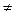s

This type of correlation arised if there were some omitted variables that were common to all equations. Both these models, in principle, be extended to include the other types of correlation. Also, in both the models it was possible to apply tests for equality of the slope coefficients before any pooling was done. For the Seemingly Unrelated Regression model first we estimated each equation separately by ordinary least square (OLS) method. After that, we obtained the estimated residuals eit. From these estimated residuals we computed the estimation of covarianceσij.

Where,σij=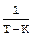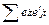Where k was the number of regression parameters estimated. After we estimatedσij, we re-estimated all the N cross-sectional equations jointly, using generalized least square method.

A number of methods were available for estimation of SUR type of models. Such as ordinary least squares (OLS) method, generalized least squares method (GLS) proposed Zellner (1962), generalized least squares method (GLS) proposedSrivastava and Giles (1987), SUR ridge regression method proposed M. A. Alkhamisi and G.Shukur(2008), optimality of least squares in the SUR Model proposed Dwivedi T. D, Srivastava V. K. (1978) etc. There were some limitations of existing methodswhichgave large MSE in case of high multicollinearity in the data set, for a large number of cross-section units the methods were not reasonable and might be affected by the common omitted variables.In this study we suggested a new method which would be able to estimate the SUR model more efficiently and the new approach might be expected to be superior to the traditional methods.

2. Literature Review

In econometrics, the seemingly unrelated regressions (SUR) or seemingly unrelated regression equations (SURE) model, proposed by Arnold Zellner in (1962) and in (1963),Stewart G. W. (1980) and Parks R. W.(1967) were a generalization of a linear regression model that consisted of several regression equations, each having its own dependent variable and potentially different sets of exogenous explanatory variables. Each equation was a valid linear regression on its own and couldbe estimated separately, which was why the system was called seemingly unrelated, although some authors suggested that the seeminglyrelated term would be more appropriate, since the error terms were assumed to be correlated across the equations. The model would be estimated equation-by-equation using standard ordinary least squares (OLS). Such estimates were consistent, however generally not as efficient as the SUR method, which amounts to feasible generalized least squares with a specific form of the variance-covariance matrix. Two important cases when SUR was in fact equivalent to OLS, were either when the error terms in fact uncorrelated between the equations (so that they were truly unrelated), or when each equation contained exactly the same set ofregressorson the right-hand-side. The SUR model could be viewed as either the simplification of the general linear model where certain coefficients in matrix Β were restricted to be equal to zero, or as the generalization of the general linear model where the regressors on the right-hand-side were allowed to be different in each equation. M. Hubert, T. Verdonck and O. Yorulmaz (Priprint) proposed a fast algorithm, FastSUR, and show its good performance in a simulation study and diagnostics for outlier detection and illustrate them on a real data set from economics. They focused on the General Multivariate Chain Ladder (GMCL) model that employs SUR to estimate its parameters. O. B. Ebukuyo, A. A. Adepoju and E. I. Olamide (2013) examined the performances of the SUR estimator with varying degree of AR(1) using Mean Square Error (MSE), the SUR estimator  performed better with autocorrelation coefficient of 0.3 than that of 0.5 in both regression equations with best MSE. Z. Zeebari and G. Shukur (2012) examined the application of the Least Absolute Deviations (LAD) method for ridge type parameter estimation of Seemingly Unrelated Regression Equations (SURE) models. M. El-Dereny and N. I. Rashwan (2011) has solved the equation in case of multicollinearity by Ridge Regression model, but not solving the SUR model in presence of multicollinearity in the data set.

3. Methodology

Different forms of generalized least squares method for the estimation of SUR model had been verified. Theoretical aspects of proposed methods such as GLS1, GLS2, and GLS3 for estimating SUR model had been described. We also showed the unbiasedness and variance property of each proposed estimator. It hadbeen found that GLS3 estimator provided less variance and less MSE compared to other proposed estimators. This study showed that the proposed method typically provided unbiased estimator with lower MSE and TMSE than traditional methods in case of severe multicollinearity. The methods are as follows:Let us assumed that there were Nresponse variables each with T observations denoted by vectors y1, y2,…... ,ytwith associated explanatory variables x1,x2,……..,xt respectively. One way of fitting these models was to treat them as unrelated multiple regression models of the form,

Yi=Xi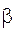+ei                                            (3.1)

Where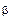was a vector of unknown regression parameters and ei was a vector of random errors with each element having variance σ2i for i=1, 2,…………….., N

Let,

X=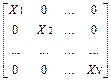,Y=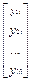,=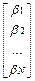, e=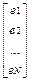By assumption,

E(ei ej´)=σijI     i, j=1,2,……..,N

Where,σij=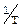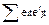andE(ee´)= Σ Ä ITwas thecovariance matrix capturing the variances and covariance of the random error terms of (3.1), then the SUR form of this model was

Y=X+e                                              (3.2)

Therefore SUR formulation of the regression models produced more efficient regression parameter estimates using proposed generalized least squares.

Some properties of proposed GLS estimators follow:

GLS1: Let us considered, the following transformation,

Y* =  (DÄIT)Y X* = (DÄIT)X e* =  (DÄIT)e

Where, D was any orthogonal matrix. [Ali, M. I. (1984)] and Ä was a kronecker product(Anderson T. W 1984). Using the above transformation the model in (3.2) be expressed as,

Y* =X*+ e*                                     (3.3)

Where, Y* and e* were NT×1 vectors, X* was an NT×n matrix.

E(e* e*´)=Σ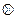IT and E(e*)=0

Then the GLS1 estimator ofin (3.1) was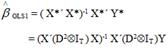(3.4)

Where, D was an orthogonal matrix.

Theorem:GLS1was an unbiased estimator of.

E (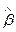GLS1) =Theorem:V(GLS1)= E[{GLS1-E()} {GLS1-E()}´]Rahman M. (2008)

=(X´(D2ÄIT)X)-1 X´(D2ÄIT)(ΣIT )(D2ÄIT)X (X´(D2ÄIT)X)-1

GLS2 :Let us considered, the following transformation,

Y* = (S-1 ÄIT) Y X* = XS-1 e* = eS-1

Using the above transformation the model in (3.2) be expressed as,

Y* =X*+ e*                         (3.5)

Where, Y* and e* were NT×1 vectors, X* was an NT×n matrix.

E (e* e*´)=ΣIT  and E(e*)=0

When Σ was known then the GLS estimator of ß in (3.5) was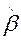GLS2 = (X*´ X*)-1 X*´ Y*

= (S-1X´XS-1)-1S-1(S-1 ÄIT)Y

When the covariance matrix Σ (Alan J. L 2004)was unknown, a feasible generalized least squares (FGLS) (Johnston J, DiNardo J. 1963, 1972 and 1984) estimator was defined by replacing the unknown Σ with a consistent estimate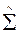was given by,=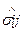=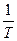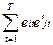Then,GLS2= (S^-1X´XS^-1)-1S^-1X´ (S^-1 ÄIT)Y           (3.6)

Theorem:GLS2 was not an unbiased estimator of.

E(GLS2)Theorem:V(GLS2)= E[{GLS2-E()} {GLS2-E()}´]

= (S^-1X´XS^-1)-1S^-1X´(S^-1 ÄIT)XS^-1 (S^-1X´XS^-1)-1

GLS3 :Again, let us considered, the following transformation,

Y* = (S-1 ÄIT) Y,    X* = (S-1ÄIT) X,    e* = (S-1ÄIT)e

Using the above transformation the model in (3.2) be expressed as,

Y* =X*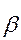+ e*                  (3.7)

Where, Y* and e*   were NT×1 vectors, X* was an NT×n matrix

E(e* e*´)=ΣIT and E(e*)=0

When Σ was known then the GLS3 estimator ofin (3.7) becameGLS3 =(X*´ X*)-1 X*´ Y*

= [{(S-1 ÄIT)X}´{(S-1 ÄIT)X}]-1{(S-1 ÄIT)X}´{(S-1 ÄIT)Y}

= (S-2X)-1S-2Y                       (3.8)

When the covariance matrix Σ was unknown a feasible generalized least squares (FGLS) estimator was defined by replacing the unknown Σ with a consistent estimatewas given by,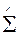=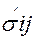=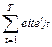Then,FGLS3=(S^-2X)-1S^-2Y                                  (3.9)

Theorem:GLS3 was an unbiased estimator of.

E (GLS3) =Theorem: V(GLS3)= E[(GLS3-) (GLS3-)´]

={X´(S^-2ÄIT)X}-1 {X´(S^-3ÄIT) X}{X´(S^-2ÄIT)X}-1

It had been found that GLS3 estimator provided less variance and less MSE compared to other proposed estimators such as GLS1 and GLS2, so that,

V (GLS3) < V (GLS2) <V (GLS1)

The results were verified using real data and simulated data. The empirical results were presented in Table 1, 2. The results were also compared with the aid of graphs.

Table 1. System-wise estimated MSEs and TMSEs for the different methods of observation, N =2 equations, T=8, 16, 32 observations.

 Types of Data Observation OLS GLS(Zellner, 1962) GLS(Srivastava and Giles,1987) Ridge Estimator for SUR Model GLS1 GLS2 GLS3 Based on Real Data T=8 MSE 0.00588 0.000012 0.000012 0.000020 0.00970 0.00000205 0.000000073 TMSE 0.13370 0.133672 0.133672 0.133672 0.13370 0.44457 0.22764 T=16 MSE 50.3009 0.044025 0.044025 0.044025 82.4437 0.00289 0.00524 TMSE 0.04873 0.048655 0.048655 0.048654 0.04873 0.06082 0.04907 T=32 MSE 203.058 0.092769 0.092769 0.092770 269.283 0.01521 0.01879 TMSE 0.02042 0.020404 0.020404 0.020404 0.02042 0.02274 0.02042 Based on Simulated Data T=8 MSE 0.55654 0.710668 0.770346 1.314708 0.75496 0.81591 0.51844 TMSE 0.00110 0.001073 0.001069 0.001076 0.00107 0.00041 0.00119 T=16 MSE 0.88032 0.765417 1.045613 0.832161 0.93289 1.11909 0.70974 TMSE 0.00052 0.000513 0.000511 0.000509 0.00051 0.00041 0.00053 T=32 MSE 0.95541 1.081081 0.775164 0.896703 0.99046 0.94065 1.03722 TMSE 0.00021 0.000212 0.000214 0.000214 0.00022 0.00041 0.00021

Table 2. System-wise estimated MSEs and TMSEs for the different methods of observation, N =3 equations, T=8, 16 observations.

 Types of Data Observation OLS GLS(Zellner, 1962) GLS(Srivastava and Giles,1987) Ridge Estimator for SUR Model GLS1 GLS2 GLS3 Based on Real Data T=8 MSE 340.019 21.70766 21.70766 21.70767 192.335 5.18659 5.18277 TMSE 0.17382 0.121873 0.121873 0.121873 0.17382 1.98253 0.28204 T=16 MSE 720.778 0.938033 0.93803 0.93803 49.4244 0.05963 0.09554 TMSE 0.06658 0.066237 0.06624 0.066237 0.06658 0.08839 0.06713 Based on Simulated Data T=8 MSE 0.81563 0.975524 1.10023 1.64572 1.24147 1.13131 0.41326 TMSE 0.00118 0.001315 0.001177 0.00159 0.00173 0.00210 0.00160 T=16 MSE 1.04617 0.897032 1.05750 0.81005 1.20330 1.22188 0.92706 TMSE 0.00082 0.000640 0.00051 0.000656 0.00054 0.00068 0.00076

4. Sources of Data

The data set was collected from a secondary sources the issues of the Federal Reserve Bulletin by G. S. Maddala (1988) : p. 364-365. Another data set was collected from the book of Introduction to Econometrics by D. N. Gujarati (1995): p. 351-353. Both the data set had severe multicollinearity and hence checked by different methods. In this paper considered variables were wage income, non-wage, the price of alternative financing to firms and production index. There were two independent variables, x1 wage income, x2 non-wage for the first set and x1 represented the price of alternative financing to firms, x2 represented industrial production index and represented firms’ expectation about future economic activity for the second set to estimate the two equations SUR model. Again we used three independent variables, x1 wage income, x2 non-wage, x3 farm income for the first set and x1 represented the price of alternative financing to firms, x2 represented industrial production index and represents firms’ expectation about future economic activity, and x3 represented average prime rate charged by banks for the second set to estimate three equations SUR model. We analyzed the data by using the software R-Language (Version-2.9.2).

5. Empirical Analysis

Algorithms for data simulation of seemingly unrelated regressions (SUR) model:

Step 1:For two equations, we had considered starting values the parameters (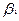,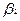) which were obtained from the real data by OLS methods. Based on these values we had simulated data for T=8, 16 and 32 observations.

Step 2:For three equation, we had considered starting values of the parameters (,,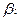) which were obtained from the real data by OLS methods. Based on these values we had simulated data for T=8 and 16 observations.

Step 3: By the similar way we repeated the simulation 1000 times and we got 1000 estimates for each parameter. Then we took mean of the simulated estimates for each parameter.

Step 4: These estimates were presented in a tabular form.

Step 5: The above procedures were repeated for two equations SUR model and three equations SUR model.

6. Statistical Results

From Table 1 it was seen that when the multicollinearity was high the MSE of two equations SUR model was larger by the methods of OLS (0.00588) and GLS (Zellner, 1962) (0.000012). The MSE obtained by the proposed method GLS3(0.000000073) was smaller than the other methods of estimation of two equations SUR model based on real data. But for the small observations (T=8) the TMSE in GLS3 method of estimation of two equations SUR model was a small amount of outsized than others based on real data. It was seen that if we increased sample size, then the MSE’s reduced but the TMSE’s were approximately equal to the others on the basis of real data. If the sample size increased more, then the MSE’s and TMSE’s declined in case of  proposed method GLS3 than other methods of estimation of two equations SUR model based on simulated data. Hence the table 1 showed that the method of GLS3 gave better estimate of SUR model in both cases of real data and simulated data with respect to MSE and TMSE criterion.

Figure 1 showed that the MSE by the proposed method GLS3 was smaller in comparison with other methods.

It was evident from Fig. 2 that the TMSE were approximately same for the different methods of estimation of two equations SUR model. From the figure we also seen that if we increased sample size, then TMSE’s increased in case of each methods for T<30 but the TMSE’s  declined for each methods for T>30,while for extremely large observations the TMSE  declined for the  methods of estimation of two equations SUR model based on different generating samples.

Table 2indicated that the MSE and TMSE of three equations SUR model were larger than the two equations SUR model  based on both  real data and simulated data.

Figure 3 showed that the MSE by the proposed method GLS3 was smaller in comparison with other methods.

Figure 4 represented that the TMSE approximately same to the different methods of estimation of two equation SUR model. From the figure it was seen that if we increased sample size, then TMSE’s increased in case of each methods for T<30 but the TMSE’s  declined for T>30 and for extremely large observations the TMSE was strictly declined by the methods of estimation of three equations SUR model based on different generating samples.

7. Results and Discussion

It had been found that the ordinary least squares (OLS), generalized least squares (GLS) by Zellner (1962), generalized least squares (GLS) by Srivastava and Giles (1987) all were unbiased, but the SUR ridge estimator by M. A. Alkhamisi and G. Shukur (2008) was not unbiased. We had computed their variances and found that SUR ridge estimator by M. A. Alkhamisi and G. Shukur (2008) provided less MSE compared to others. We had also discussed multicollinearity, causes of multicollinearity, consequences, detection and removal methods of multicollinearity in brief.MSE and TMSE criterion had been used to measure the goodness of SUR estimators. We described theoretical concepts of our proposed methods viz. GLS1, GLS2 and GLS3 for estimating SUR model for two and three equation. The proposed estimators were mainly defined on the basis of transformation or modification made in variables/matrix.

The simulation results supported the hypothesis that the number of equations, the number of observations per equation, the correlation among explanatory variables and equations were the main factors that affected the inferential properties of SUR estimators. The fitness of the models were verified to the real data and simulated data. The goodness of the proposed models had been computed in terms of MSE and TMSE.

The results showed that the MSE of GLS3 of the SUR estimator was consistently lower than the other existing estimators. Therefore, the GLS3 estimator performs better than other estimators when the errors were correlated between the equations and this could be considered as the best estimator of SUR model.

8. Conclusion

This study provided an approach to fitting SUR models when faced with some difficulties. Several methods of handling these were explored here and the simple approach of applying to estimate the SUR model by conditioning on all observations and iterating until estimates GLS3 method was computationally efficient and reasonably accurate.

Finally, under certain conditions we might be suggested GLS3 as one of the good estimators to estimate the SUR (seemingly unrelated regression) model in the presence of high multicollinearity. We also suggested that the orthogonal transformation (GLS1) was less efficient to estimate the SUR model. Our study concluded that we would use our proposed estimator GLS3  in any type of real data (except time series data) for the best fitting of SUR model in case of severe multicollinearity.

Hence the proposed method (GLS3) could be gained in estimator accuracy to other methods for small and large sample observations in terms of bias MSE and TMSE criteria.

The practical applications of the seemingly unrelated regression (SUR) model where the proposed method of estimation can be applied in order to obtain better forecasting through efficient estimation of parameters involved are mentioned below:

i.        SUR model may be used to predict or forecast the total commercial loan on different causes such as average prime rate changed by bank, bank rate, total bank deposits etc.

ii.        SUR model can be applied to an environmental situation with missing data and censored values.

iii.        SUR model may be more appropriate to predict farm’s ability in meeting their current and anticipated obligations in the next 12, 9 and 3 months etc.

iv.        SUR model may be applied for any type of simultaneous regression equations where their error terms are highly correlated.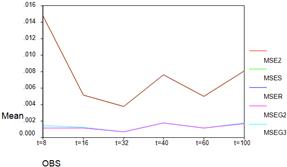Figure 1. MSE’s among different methods of estimation of two equations of SUR model.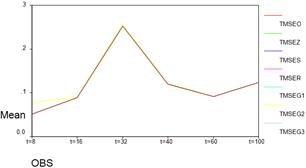Figure 2. TMSE’s among different methods of estimation of two equations of SUR model.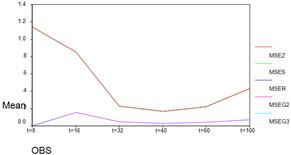Figure 3. MSE’s among different methods of estimation of three equations of SUR model.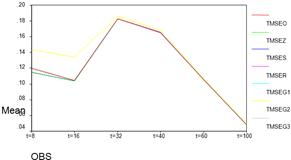Figure 4. TMSE’s among different methods of estimation of three equations of SUR model.

References

1. Alan J. L. 2004.Matrix Analysis for Scientists and Engineers. Society for Industrial and Applied Mathematics: 139-140.
2. Ali M. I. 1984. Matrices and Linear Transformations. International Student Edition: 45-212.
3. Alkhamisi M. A, Shukur  G. 2008. Developing Ridge Parameters for SUR Model,Communications in Statistics - Theory and Methods,80:544-564.
4. Anderson T. W. 1984.AnIntroduction to Multivariate Statistical Analysis.John Wiley and Sons, Inc., New York, Second edition:675.
5. Dereny M. El,  Rashwan N. I. 2011. Solving Multicollinearity Problem Using Ridge Regression Models. Int. J. Contemp. Math. Sciences, 6:585 – 600.
6. Dwivedi T. D, Srivastava V. K. 1978.Optimality of least squares in the seemingly unrelated regression equation model.Journal of Econometrics, 7: 391-395.
7. Gujarati D. N. 1995. Basic Econometrics. McGraw-Hill, New York. Third edition: 826. Hubert M, Verdonck T, O. Yorulmaz O. Preprint.
8. Johnston J, DiNardo J. 1963, 1972 and 1984. Econometric Methods. McGRAW-HILL INTERNATIONAL EDITIONS Fourth edition: 531.
9. Maddala G. S. 1988. Introduction to Econometrics, Macmillan international editions: 364-365.
10. Ebukuyo O. B, Adepoju A. A, Olamide E. I. 2013.Bootstrap Approach for Estimating Seemingly Unrelated Regressions with Varying Degree of Autocorrelated Disturbances.Journal of Progress in Applied Mathematics,5:55-63.
11. Parks R. W.1967. Efficient Estimation of a System of Regression Equations WhenDisturbances are Both Serially and Contemporaneously Correlated.Journal of theAmerican Statistical Association 62:500-509.
12. Rahman M. 2008. Basic Econometrics, Theory and Practice, The University Grants Commission Dhaka. First edition.
13. Srivastava V, Giles D. 1987.Seemingly Unrelated Regression Equations Models. New York: Marcel Dekker.
14. Stewart G. W. 1980. The Efficient Generation of Random Orthogonal Matrices with an Application to Condition Estimators. SIAM J. Numer. Anal.17 (3): 403–409.
15. Zeebari Z, Shukur G. 2012. On the Least Absolute Deviations Method for Ridge Estimation of SURE Models.In:Communications in Statistics - Theory and Methods, ISSN 0361-0926, E-ISSN 1532-415XArticle in journal.
16. Zellner A. 1962. An efficient method of estimating seemingly unrelated regressions and tests for aggregation bias.Journal of the American Statistical Association,57:348-368.
17. Zellner A. 1963. Estimators for seemingly unrelated regression equations: some exact finite sample results.Journal of the American Statistical Association, 58: 977-992.

 Contents 1. 2. 3. 4. 5. 6. 7. 8.
Article ToolsAbstractPDF(270K)